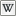# Category:LSL Float

 LSL Portal

The LSL "float" type is afloating point data type that uses 32 bit inIEEE-754 form. If a number is written with a decimal point in LSL, then it is taken to be a float.

The valid range is 1.401298464E-45 to 3.402823466E+38

Floats can be specified in scientific notation such as 2.6E-5.

If a function requires a float as a parameter, and the number is an integer (e.g. 5), you can add the .0 to clearly indicate it's a float, but omitting the .0 is equally valid and actually saves bytecode space in the compiled code.

When dividing 2 constants, defining them as floats will avoid the chance of unwanted rounding down. Better still, do the math on your calculator and save the server some cycles.

## Examples

```float min = 1.175494351E-38;
float max = 3.402823466E+38;
float sci = 2.6E-5;
float sci_a = 2.6E+3;
float sci_b = 2.6E3;
float sci_c = 26000.E-1;
float f = 2600;//implicitly typecast to a float
float E = 85.34859;
float cast = (float)"42";//explicit typecast to a float
float Infintity = (float)"inf"; //-- may be negative, will cause a math error if evaluated in LSO, see 'caveats' below
float NotANumber = (float)"nan"; //-- may be negative, will cause a math error if evaluated in LSO, see 'caveats' bleow
```

## Useful Snippets

If you need to validate an arbitrary float without limitations then the following function is ideal:

```integer isValidFloat(string s) { return (string)((float)s) != (string)((float)("-" + llStringTrim(s, STRING_TRIM_HEAD))); }
```

However, the following is more efficient, but comes with the noted caveats. If these are not an issue to you then it is the recommended option, particularly under Mono:

```integer isValidFloat(string s) { return (float)(s + "1") != 0.0; }
```

Caveats:

• LSO-LSL scientific notation with an exponent greater than 38 will fail (throw a Math Error). Mono is unaffected as it supports `infinity`
• Under both Mono and LSO-LSL you may find strange results if dealing with strings containing more than 9 decimal places. Remember that string casting in LSL only gives up to 6 so is safe, and human input is rarely going to be that accurate, plus values that small are not usually all that useful.
• Due to the limited precision with which floats are stored, not all integer values can be accurately held in a float. Integers above 16,777,216 are rounded down to the nearest even number; above 33,554,432 to the lower multiple of 4 etc.
• "nan" (not-a-number), "inf" (infinity) and their negatives are special text values that can be cast from a string (with any leading spaces or trailing characters). those values will cause a math error when the variable is evaluated in LSO. If you are parsing user data, by casting a string to a float, use the following code (replacing vStrDta with your string variable name) see SVC-6847:
```(float)llList2String( llParseStringKeepNulls( llToLower( llStringTrim( vStrDta, STRING_TRIM ) ), ["inf", "nan"], [] ), 0 )
```

However, both of the above snippets will stop validating at the first character that is not valid in a float string. The following code rigorously validates the whole of a string to ensure it represents a float (and nothing else), at the cost of speed and memory footprint.

```// Validate a string containing a float value
// Does not handle scientific notation, or hex floats (!!)
// After all, this is designed for 95% of likely human entered data

integer  ValidateSimpleFloat(string sin)
{
sin = llToLower(sin);
// Avoid run time fail (for lslEditor at least) if string looks remotely like scientific notation
if (llSubStringIndex(sin, "e") != -1)   	return FALSE;
list temp = llParseStringKeepNulls(sin, ["."], [] );
string subs = llList2String(temp, 0);
if ( (string) ( (integer) subs) != subs)    return FALSE;
if ( (temp != []) > 2)                      return FALSE;
if ( (temp != [])== 2)
{
subs = llList2String(temp, 1);    // extract the decimal part
// must have no sign after DP, so handle first decimal discretely
string first = llGetSubString(subs, 0, 0);
if ( (string) ( (integer) first) != first)     return FALSE;
if ( (string) ( (integer) subs)  != subs)      return FALSE;
}
return TRUE;
}
```

## Float-to-String

There are several ways to convert a float to a string. The first of which is to typecast it to a string `(string)(1.0)`. This however has the disadvantage of rounding and being limited to six decimal places. Several functions have been written to provide more options. They fall into two categories, lossless and lossy.

Lossy functions
Name inf/nan Rounding Truncation Notes
Typecast Yes Yes No `(string)float_value`
Mono only gives 6 digits of precision.
Format Decimal No Yes No
Float2String No Yes Yes
Lossless functions
Name Speed Reversible inf/nan support PI Notes
Float2Hex Fast `(float)` No 0x6487ED5p-25 Since the output is in the Hexadecimal Scientific Notation, it's not really human readable.
Float2Sci Slow `(float)` No 3.1415925 Useful when you want the result to be lossless but also human readable, comes at the cost of speed.
FUIS Fastest SIUF No "QEkP2g" Not at all human readable. Guarantied to always use six characters.
• Infinity is only accessible in Mono.

## Subcategories

This category has only the following subcategory.

## Pages in category "LSL Float"

The following 8 pages are in this category, out of 8 total.